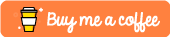# Creating Various Filters With OpenCV/Python

## Introduction

Hello! I recently decided to add more filters to my previous cartoon filter example which can be found here:

https://dev.to/ethand91/cartoon-filter-using-opencv-and-python-3nj5

This post continues from the above post so I won't be going over creating the virtual environment etc.

Well then lets add some more cool filters! 😎

## HDR Filter

The HDR effect filter is simple to implement we just use the detailEnhance method:

``````def HD (image):
hdrImage = cv2.detailEnhance(image, sigma_s = 12, sigma_r = 0.15)

return hdrImage
``````

sigma_s controls how much the image is smoothed and sigma_r is important to preserve edges while smoothing the image.## Pencil Sketch GreyScale/Color Filter

This is another easy to implement filter as opencv already has a method that can do this for us. This method returns both the greyscale image and the color image, here I am returning the color version but feel free to try out the greyscale image too.

``````def pencil (image):
sk_gray, skColor = cv2.pencilSketch(image, sigma_s = 60, sigma_r = 0.07, shade_factor = 0.1)

return skColor
``````## Sepia Filter

The sepia filter lets us apply a brown, calm effect to images. To do this we convert to float to prevent loss, transform the image and then finally normalizing the values.

``````def sepia (image):
# Convert to float to prevent loss
sepiaImage = np.array(image, dtype = np.float64)
sepiaImage = cv2.transform(sepiaImage, np.matrix([[0.272, 0.543, 0.131], [0.349, 0.686, 0.168], [0.393, 0.769, 0.189]]))
sepiaImage[np.where(sepiaImage > 255)] = 255
sepiaImage = np.array(sepiaImage, dtype = np.uint8)

return sepiaImage
``````## Sharpen Filter

To sharpen the image we will use the filter2D method and apply the following kernel.

``````def sharpen (image):
kernel = np.array([[-1, -1, -1], [-1, 9.5, -1], [-1, -1, -1]])
sharpenedImage = cv2.filter2D(image, -1, kernel)

return sharpenedImage
``````## Brightness Filter

To adjust the image's brightness we will use the convertScaleAbs method.

``````def brightness (image, betaValue):
brightImage = cv2.convertScaleAbs(image, beta = betaValue)

return brightImage
``````We can also use this filter to make the image darker by passing a negative value to beta.## Greyscale Filter

Greyscale filter is another easy filter to implement wi just use the cvtColor method.

``````def grayScale (image):
grayImage = cv2.cvtColor(image, cv2.COLOR_BGR2GRAY)

return grayImage
``````## Invert Filter

Another easy filter to implement we just invert the pixel values using the bitwise_not method.

``````def invert (image):
invertedImage = cv2.bitwise_not(image)

return invertedImage
``````## Saving the images

Once we have all the filtering methods defined it would be a shame not to use them. Edit the main method to include the following:

``````cartoonImage = cartoonize(image)
invertedImage = invert(image)
grayImage = grayScale(image)
brightImage = brightness(image, 60)
darkerImage = brightness(image, -60)
sharperImage = sharpen(image)
sepiaImage = sepia(image)
pencilImage = pencil(image)
hdrImage = HD(image)
``````

Finally we can write the output to file with the following, append them to the main method:

``````cv2.imwrite("output.jpg", cartoonImage)
cv2.imwrite("inverted.jpg", invertedImage)
cv2.imwrite("grayscale.jpg", grayImage);
cv2.imwrite("brighter.jpg", brightImage)
cv2.imwrite("darker.jpg", darkerImage)
cv2.imwrite("sharper.jpg", sharperImage)
cv2.imwrite("sepia.jpg", sepiaImage)
cv2.imwrite("pencil.jpg", pencilImage)
cv2.imwrite("hdrImage.jpg", hdrImage)
``````

Done!

Feel free to change the values etc, to see what different effects you can make. 🥳

## Conclusion

Here I have shown how to apply various filters to an image, using opencv's methods it wasn't too hard to implement.

I will make another post if I manage to find more interesting/fun filters. 🥸

The repo can be found here: https://github.com/ethand91/opencv-cartoon-filter

Like me work? I post about a variety of topics, if you would like to see more please like and follow me. Also I love coffee.